Error calibration

The first step to handling errors is to quantify them. Make sure that your data’s “dilution of precision” (DOP) and error columns import correctly into ctmm. In the following wood turtle dataset, we have some calibration data and a turtle track. Note that the calibration data must be collected from the same model device as the tracking data, and their data formatting bust be similar enough that the same location classes are detected by as.telemetry.

library(ctmm)
data(turtle)
names(turtle[]) # data are not yet calibrated, but HDOP and location class is present
##  "t"     "HDOP"  "class" "x"     "y"
names(turtle) # two calibration datasets and two turtle datasets
##  "60s"  "90s"  "F231" "F403"
plot(turtle[1:2],col=rainbow(2)) # calibration data only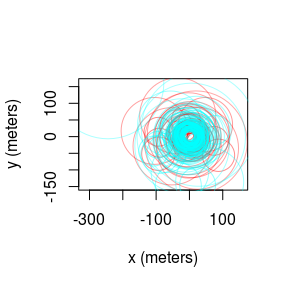The uere command is used to estimate the UERE parameter(s) from calibration data. Do not use this command on animal tracking data.

UERE <- uere.fit(turtle[1:2]) # only using calibration data
summary(UERE)
## , , horizontal
##
##          low        ML      high
## 2D 22.786528 29.598802 36.397467
## 3D  6.477391  6.946024  7.414237

For GPS data, the UERE will typically be 10-15 meters. Here we have two location classes, “2D” and “3D”, which have substantially different UERE values. The UERE can then be assigned to a dataset with the uere()<- command.

uere(turtle) <- UERE
names(turtle[]) # now the data are calibrated, as VAR is present
##  "t"      "HDOP"   "class"  "x"      "y"      "VAR.xy"
plot(turtle[],error=2) # turtle plot with 95% error discs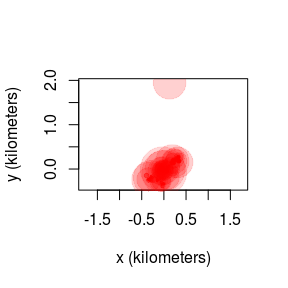If you have ARGOS or e-obs data, they will import with calibration already applied to the horizontal dimensions, but not the vertical dimension. These data only need their vertical errors calibrated.

data(coati)
names(coati[]) # VAR already present
##   "timestamp" "longitude" "latitude"  "t"         "HDOP"
##   "VAR.xy"    "class"     "z"         "VDOP"      "speed"
##  "heading"   "SDOP"      "x"         "y"         "vx"
##  "vy"
plot(coati[],error=2) # coati plot with 95% error discs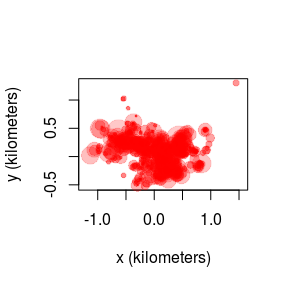Error model selection

Not all GPS devices provide reliable DOP values and sometimes it is not obvious what error information will prove to be the most predictive. Generally speaking, as.telemetry will attempt to import the “best” column from among those that estimate error, DOP value, number of satellites and fix type, inlcuding timed-out fixes (see the timeout argument in help(as.telemetry)). Researchers may want to import their data with different error information, run uere.fit for each error model, and then select among the candidate models. Here we do this by comparing the turtle calibration data with and without HDOP values.

First, we consider whether or not the HDOP and location class information are informative.

t.noHDOP  <- lapply(turtle,function(t){ t$HDOP <- NULL; t }) t.noclass <- lapply(turtle,function(t){ t$class <- NULL; t })
t.nothing <- lapply(turtle,function(t){ t$HDOP <- NULL; t$class <- NULL; t })

In other cases, manipulation will need to be performed before importing, so that as.telemetry can format the telemetry object properly. Running uere.fit now results in errors calibrated under the assumption of homoskedastic errors.

UERE.noHDOP  <- uere.fit(t.noHDOP[1:2])
UERE.noclass <- uere.fit(t.noclass[1:2])
UERE.nothing <- uere.fit(t.nothing[1:2])

We can now apply model selection to the UERE model fits by summarizing them in a list.

summary(list(HDOP.class=UERE,class=UERE.noHDOP,HDOP=UERE.noclass,homoskedastic=UERE.nothing))
##                  ΔAICc  Z[red]²
## HDOP.class      0.0000 3.741176
## HDOP          286.5747 6.180647
## class         418.5174 5.844317
## homoskedastic 637.2580 7.401718

We can see that the combination of location class and HDOP values yield the best error model, both in terms of AICc and in terms of reduced Z squared. Reduced Z squared is a goodness-of-fit statistic akin to reduced chi squared, but designed for comparing error models.

We had two calibration datasets, so we can also see if there is any substantial difference between the two GPS tags. We do this by making a list of individual UEREs to compare to the previous joint UERE.

UERES <- lapply(turtle[1:2],uere.fit)
summary(list(joint=UERE,individual=UERES))
##               ΔAICc  Z[red]²
## joint      0.000000 3.741176
## individual 3.314758 3.738773

In this case, performance of the individualized and joint error models are comparable, and AICc selects the joint model.

Outlier detection

Now we come to the task of identifying outliers. The outlie function uses error information to estimate straight-line speeds between sampled times and distances from the bulk of the data.

outlie(turtle[]) -> OUT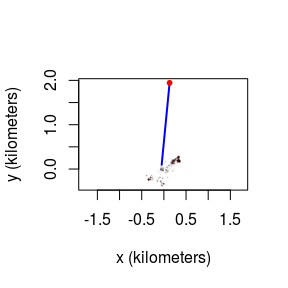High-speed segments are colored in blue, while distant locations are colored in red. More emphasis is placed on the more extreme locations in the outlie plot. Visually we can see at least one outlier in the wood turtle data. The output of outlie also contains the error-informed speed and distance estimates (in SI units) used to generate the plot.

plot(OUT)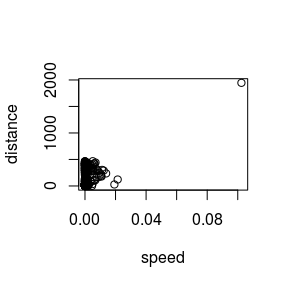A sustained speed of 0.1 m/s is not biologically implausible for a wood turtle, but this location is highly suspicious, both in terms of speed and lack of proximity. After removing the outlier, we can check the data again.

BAD <- which.max(OUT$speed) turtle[] <- turtle[][-BAD,] outlie(turtle[]) -> OUT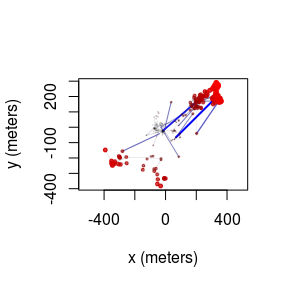Datasets may have multiple outliers. In pathological situations, there may be no clear separation between the normative data and the outliers. This necessitates a better error model, either by improving inadequate (or absent) HDOP estimates or by employing a heavier tailed error distribution (not yet supported). Variograms and model selection Variograms If we were working with ARGOS data or high resolution GPS data on a small animal, then we can get a “nugget” effect in the variogram that looks like an initial discontinuity at short time lags.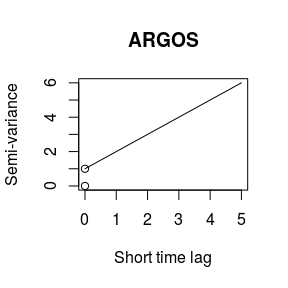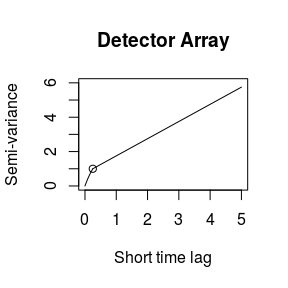The height of this initial discontinuity corresponds to the variance of uncorrelated location errors. The second plot is the kind of initial discontinuity one has with detector array data. The end of the (slope) discontinuity is highlighted with a circle. This discontinuity is smooth because the movement and detection are correlated. The height of this initial discontinuity is also (at least roughly) the variance of the location errors. Model fitting Because of some convenient mathematical relations, fitting with telemetry errors involves numerically fitting 1-4 more parameters and is, therefore, slower and less reliable at converging on the MLE than fitting without telemetry error. Therefore, by default, telemetry error is not turned on in ctmm models (error=FALSE). Furthermore, in cases with an error model, ctmm.select() is safer to use than direct applicaiton of ctmm.fit(), because ctmm.select() will start with the simplest “compatible” model and then consider further model features that require numerical optimization in stepwise fashion. Fitting with calibrated data For calibrated errors, we have to set error=TRUE in the model guess to fit with telemetry error accounted for. # automated guestimates with circular covariance and calibrated errors GUESS <- ctmm.guess(turtle[],CTMM=ctmm(error=TRUE),interactive=FALSE) # the beta optimizer is more reliable than the default optimizer control <- list(method='pNewton') # stepwise fitting # CRAN policy limits us to 2 cores FIT <- ctmm.select(turtle[],GUESS,control=control,trace=TRUE,cores=2) ## * Fitting model OUF isotropic error. ## * Fitting models OUF anisotropic error. ## * Fitting models OU anisotropic error, OUf anisotropic error. ## * Fitting models IID anisotropic error, OU isotropic error. summary(FIT) ##$name
##  "OU anisotropic error"
##
## $DOF ## mean area speed ## 14.90651 28.89103 0.00000 ## ##$CI
##                          low        ML      high
## area (hectares)    13.365481 19.973559 27.887949
## τ[position] (days)  3.263703  5.272276  8.516981

trace=TRUE allows us to see the models considered. verbose=TRUE would save each model.

Fitting with uncalibrated data

Fitting with unknown errors is a method of last resort. We have to provide an initial guess for error, which will be the device’s UERE. error=10 is usually a good guess for GPS data. Unlike uere.fit, ctmm.fit is not yet coded to use the location classes.

# delete UERE information
uere(turtle) <- NULL
# toss out 2D locations for now
turtle <- lapply(turtle,function(t){ t[t$class=="3D",] }) The only difference is that you have to provide an initial guess for the UERE. Otherwise, the steps are the same. # cheat and use previous fit as initial guess GUESS$error <- 10 # 10 meter error guess
# fit parameter estimates
FIT <- ctmm.select(turtle[],GUESS,control=control,trace=TRUE,cores=2)
## * Fitting model OUF isotropic error.
## * Fitting models OUF anisotropic error.
## * Fitting models OU anisotropic error, OUf anisotropic error.
## * Fitting models IID anisotropic error, OU isotropic error.
summary(FIT)
## $name ##  "OU anisotropic error" ## ##$DOF
##     mean     area    speed
## 13.73097 26.50325  0.00000
##
## \$CI
##                          low        ML      high
## area (hectares)    12.735711 19.408989 27.465700
## τ[position] (days)  3.401556  5.758166  9.747442
## error (meters)      0.000000  8.761909 66.871825

Here, fitting the UERE simultaneously with the movement model produced a consistent result. This will not always be the case.